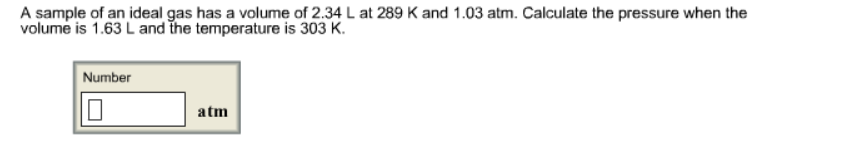# Problem: A sample of an ideal gas has a volume of 2.34 L at 289 K and 1.03 atm. Calculate the pressure when the volume is 1.63 L and the temperature is 303 K.

###### FREE Expert Solution
92% (281 ratings)###### Problem Details

A sample of an ideal gas has a volume of 2.34 L at 289 K and 1.03 atm. Calculate the pressure when the volume is 1.63 L and the temperature is 303 K.